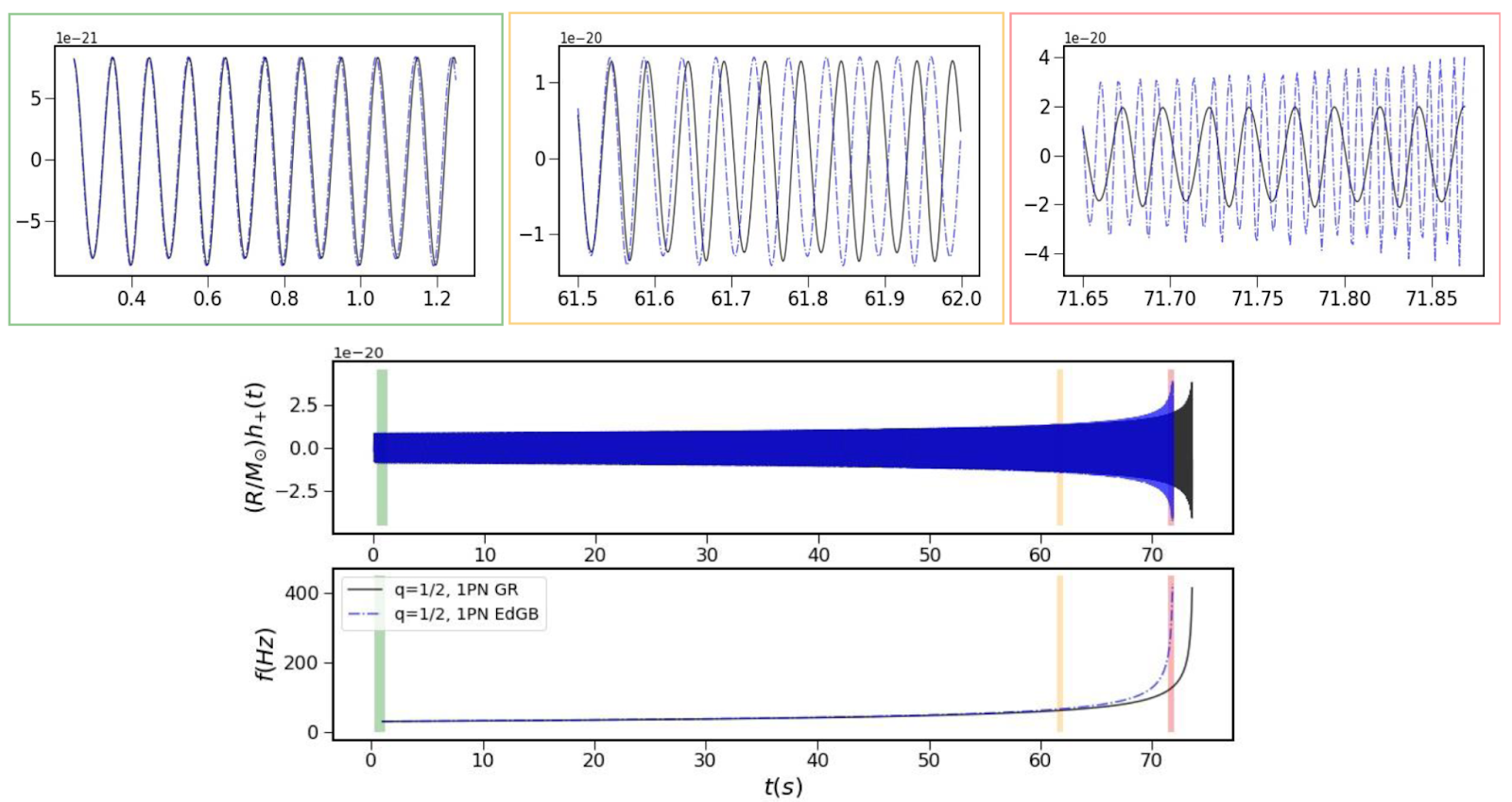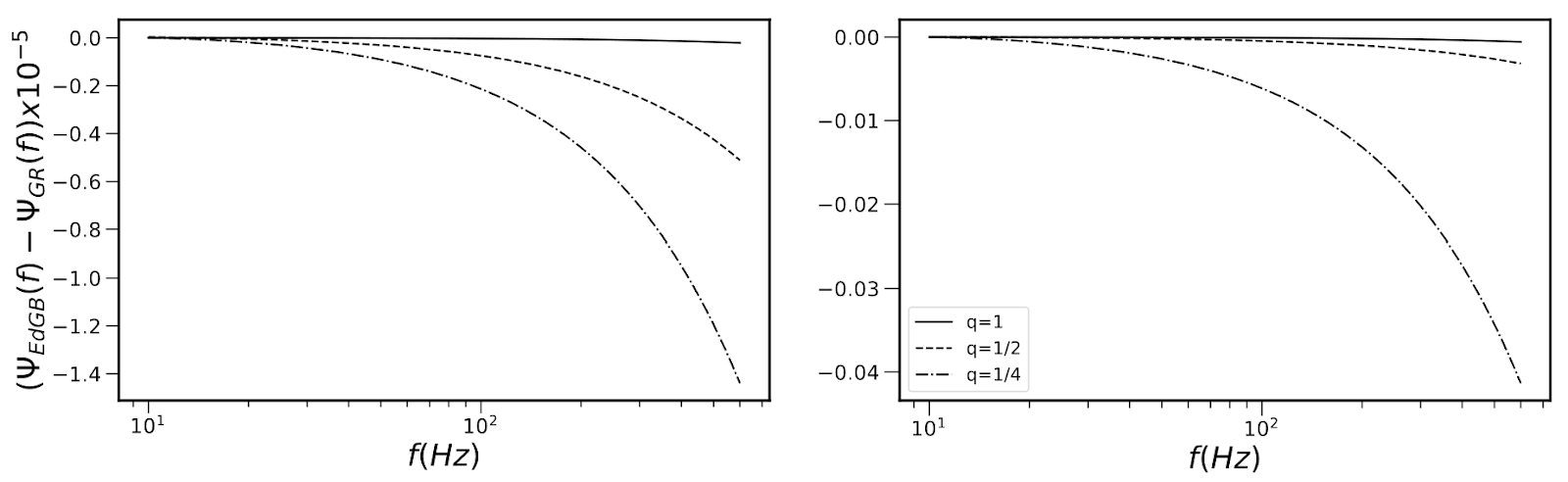# How to use gravitational waves to test if Einstein was right

This bite was written by Iris Gemeren: I’m Iris van Gemeren, currently in my second year of the Masters Theoretical Physics at Utrecht University in the Netherlands. My master research focuses on the search of hints of modified gravity theories with gravitational waves. My other interest include cosmology, dark matter, physics education and looking through a telescope once in a while! When I’m not physics’ing I like to read, write or go climbing with my friends.

Title: Nonlinear curvature effects in gravitational waves from inspiralling black hole binaries

Authors: Banafsheh Shiralilou, Tanja Hinderer, Samaya M. Nissanke, N ́estor Ortiz and Helvi Witek

First Author’s institution: GRAPPA, Anton Pannekoek Institute for Astronomy and Institute of High-Energy Physics, University of Amsterdam, Science Park 904, 1098 XH Amsterdam

Status: Published in Physical Review D [open access on the arxiv]

Gravitational waves are the key to understanding one of the most extreme environments in the universe which can possibly improve our current theory of gravity. To this day our description of how gravity works is given by Einstein’s theory of general relativity (GR). It explains how the curvature in the fabric of space and time causes the earth to rotate around the sun, light to bend around a black hole and you not floating out of your chair while reading this. It also explains why two blackholes that rotate around each other produce gravitational waves. As blackholes are very heavy, they curve spacetime a lot. When inspiralling and merging they cause ripples in the spacetime fabric that wave out at the speed of light. Astronomers are able to measure these waves, which can be compared to the wave functions calculated by GR.This gives the ultimate check if the theory describes gravity well, even in these extreme merger events.

## New theory of gravity?

General relativity works very well to describe gravity. However, in extreme situations like inside a black hole or on very small scales, the theory fails. To solve the latter, one has to combine General relativity with the theory of Quantum mechanics. How to do this is still an open question. Therefore, it seems that the theory of GR is incomplete. A lot of other theories are suggested to improve on General relativity. The goal of the authors of today’s paper is to describe how to use the gravitational waves to test an improved version of GR.

To start off, they took another promising theory of gravity that adds extra terms to the equations of GR. The theory is called scalar Gauss-Bonnet (GB) gravity, and it actually originates from a quantum mechanical description of gravity. Hence it can be used to describe gravity on these small scales, solving one of the problems mentioned before. They started with the field equations from the GB theory which describe the relation between the curvature of spacetime and the distribution of matter. With these equations one can calculate the form of the ripples in your spacetime; the gravitational waves. In this calculation the authors also use the Post Newtonian approximation, which assumes that the velocities of the black holes are not too high compared to the speed of light and the gravity is not too strong. You need this approximation as the full set of equations is very difficult to solve.

## General relativity vs. scalar Gauss-Bonnet gravity

After the calculation they plotted the waves in the GB theory and also in GR to see the differences, see the figure 1.Figure 1: The top three plots show the wave functions against the frequency calculated in GB theory (blue dashed line) and in GR (black solid line) for gravitational waves produced by two blackholes inspiralling and merging, one half as heavy as the other. The three different colours correspond to three moments during the event, beginning (green), end inspiralling (yellow) and merging (red). The bottom two plots show how (a component of) the amplitude and the frequency change during the event in time.

The top three plots show that the later in the event (orange and red respectively) the more the amplitude and phase of the waves of the GB case differ from the GR case. This means that when comparing these waveforms with the waveforms from real data at a later moment, one should be able to distinguish if the data fits better for the GR case or the GB case.

Lastly the authors also looked at the phase evolution of the gravitational waves explicitly, to see what the effect is of different mass ratios of the two blackholes. The phase is a quantity describing how many cycles of the wave have gone by. The phase evolution is then given by the change of this phase over time. They also looked at the strength of the coupling. Coupling is the measure of how strong the extra terms in GB theory with respect to GR contribute. In other words are these terms multiplied with a big number, which will give a strong contribution, or with a small number, which gives a weak contribution. The larger the coupling, the stronger the contribution and the greater GB differs from GR. They plotted the difference in the phase against the frequency, see figure 2.Figure 2: The difference in the phase of the gravitational waves in GB theory and GR plotted against the frequency. The solid line is for an event with two equally heavy blackholes, for the dotted line one is half as heavy as the other and for the dashed line one is a quarter as heavy as the other. The left plot is for a coupling of 0.03 and the right plot is for a coupling of 0.005.

These plots show that for more unequal masses of the blackholes, the phase difference becomes larger. The difference between GR and GB should therefore be more clear for systems with unequal mass. Furthermore, the difference in phase is larger for a stronger coupling. Physicists do not know yet how large the coupling of these terms is. By comparing these templates with real data they could constrain the value of the coupling by finding the best match.

All in all the authors provided templates of how the gravitational waves look like for a merger of two black holes in GB theory. They can be used to compare with real data to see if it describes the waves better than with GR. As they saw differences in the phase and waveforms of the waves in GB theory compared to the GR case, this may be in reach. Additionally, this work could help constrain the strength of the coupling for GB theory. Hopefully this can give us more hints to the true nature of the mysterious force of gravity.

Featured Image Credit: Edited figure 1 of original article and Caltech JPL
Editor: Abby Waggoner